# Introduction

One of the five tools which we will use in this course are Taylor series. These have been covered in your Calculus courses, so this topic is a general overview. For further help, see your calculus text.

# Theory

In your calculus course, you have probably seen Taylor series written as

where x0 is a point around which we are expanding, and x is some point in the neighbourhood. In this course, we will rewrite this as:

where x is some point around which we are expanding the Taylor series and h is a small value. To recall some terminology, the approximation f(x + h) = f(x) is called a zeroth-order Taylor-series approximation, while f(x + h) = f(x) + f(1)(x) h is a first-order Taylor-series approximation.

To view the usefulness of Taylor series, Figures 1, 2, and 3 show the 0th-, 1st-, and 2nd-order Taylor series approxiations of the exponential function f(x) = ex at x = 0. While the approximation in Figure 1 becomes poor very quickly, it is quite apparent that the linear, or 1st-order, approximation in Figure 2 is already quite reasonable in a small interval around x = 0. The quadratic, or 2nd-order, approximation in Figure 3 is even better.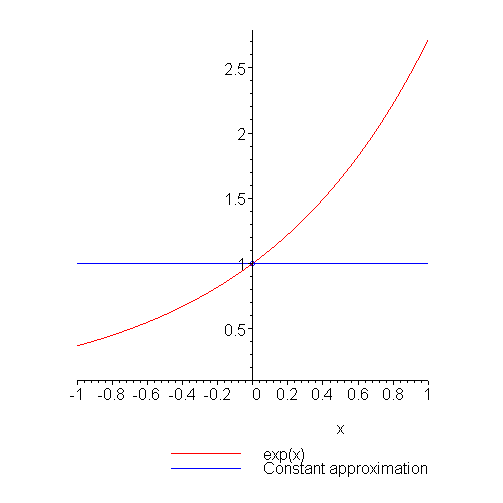Figure 1. The zeroth-order Taylor series approximation of ex around x = 0.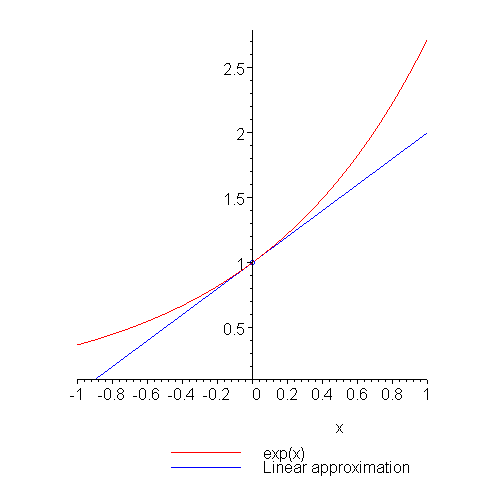Figure 2. The first-order Taylor series approximation of ex around x = 0.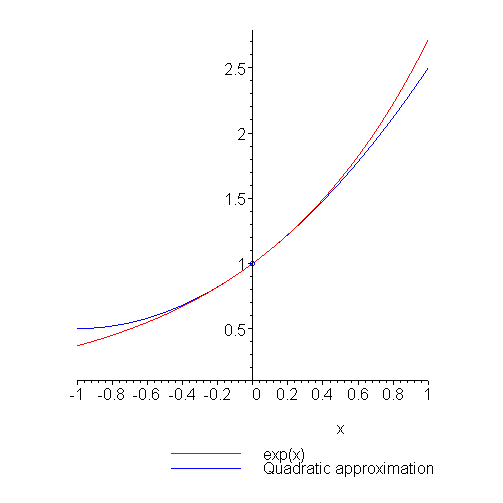Figure 3. The second-order Taylor series approximation of ex around x = 0.

We will use Taylor series for two purposes:

1. To linearize a system, using the 1st-order Taylor-series approximation, and
2. To perform error analysis on numerical method.

The most useful feature is that in many cases we can use a 1st-order Taylor series, that is, a linear polynomial, to approximate a function locally. This is a result of the observation that any differentiable function, locally, looks like a straight line. To observe this, take a look at the HOWTO on differentiable functions. Thus, if we are in the neighbourhood of a point and we have some knowledge as to its Taylor series (from our model) then we may successfully approximate the value of points on the curve in that given neighbourhood.

# Examples

To show how good Taylor series are at approximating a funciton, Figures 4 and 5 show successively higher and higher Taylor series approximations, starting with the zeroth order Taylor series approximation, of the function f(x) = sin(x) around the point x = 1.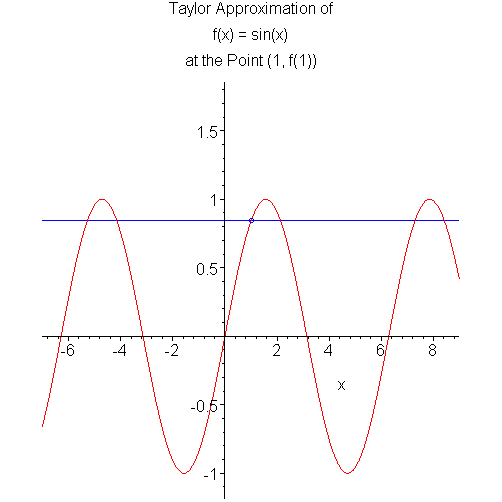Figure 4. The 0th- through 20th-order Taylor series approximations of f(x) = sin(x) around x = 1.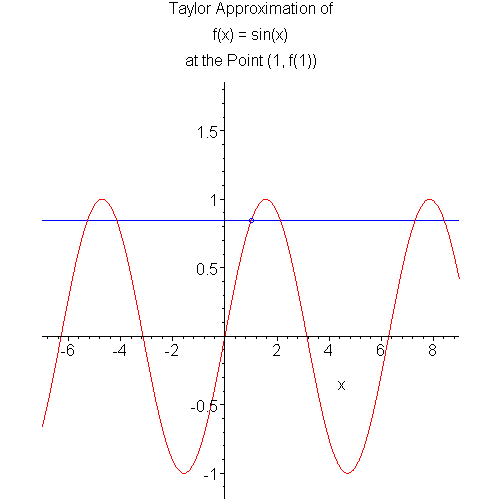Figure 5. A cummulative plot of the 0th- through 20th-order Taylor series approximations of f(x) = sin(x) around x = 1.

# HOWTO

Given a function f(x) and point x, we calculate the nth-order Taylor series around x by evaluating the function and its first n derivatives at x and writing down:

If you now wish to approximate the value of f(x + h), simply evaluate the above series with the given value of h.

The error associated with the above Taylor approximation is:

where ξ ∈ [x, x + h] (or [x + h, x] if h is negative).

In most cases, engineers use linear approximations:

If h is small (e.g., 0.001), then h2 will be very small (0.000001), and therefore, unless the second derivative is very large, the error will be resonably small.

# Example 1

Rewrite the Taylor series

f(x) = f(x0) + f(1)(x0)(x - x0) + 1/2 f(2)(x0)(x - x0)2 + 1/6 f(3)(x0)(x - x0)3

for f(x0 + h) where h = x - x0.

Answer: f(x0 + h) = f(x0) + f(1)(x0)h + 1/2 f(2)(x0)h2 + 1/6 f(3)(x0)h3

# Example 2

Find the 1st-order Taylor series approximation to sin(1.1) given the Taylor series of sin(x) around x = 1.

Answer: sin(1.1) ≈ sin(1) + cos(1) ⋅ 0.1 = 0.89550.

# Example 3

Find the 2nd-order Taylor series approximation to sin(1.1) given the Taylor series of sin(x) around x = 1.

Answer: sin(1.1) ≈ sin(1) + cos(1) ⋅ 0.1 - 0.5 sin(1) ⋅ 0.12 = 0.89129.

# Question 1

Approximate sin(1.1) using a first-order Taylor series expanded around x = 1. What is the relative error of this answer?

# Question 2

What is the bound on the error of using a first-order Taylor series expanded around x = 0.5 for the function f(x) = ex when estimating ex for x = 0 and x = 1?

Answer: [0.125, 0.125e0.5] ≈ [0.125, 0.20609] and [0.125e0.5, 0.125e] ≈ [0.20609, 0.33979].

(The actual errors are 0.17564 and 0.24520, both of which fall within the bounds found above.)

# Matlab

Taylor series are not applicable to Matlab. They must be computed manually and programmed.

# Maple

Finding Taylor series in Maple is easier than opening your Calculus text. The first argument is the function, the second is the variable and the point around which the Taylor exapnsion is to take place, and the third is the desired order.

```series( sin(x), x = 0, 10 );
series( cos(x), x = 1, 5 );
```